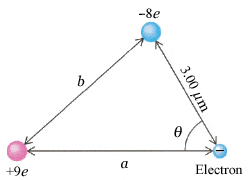# Forces Between Charges

thst1003
An electron is near a positive ion of charge +9e and a negative ion of charge −8e (see the figure below). (Take a = 5.23 µm, b = 4.28 µm, and θ = 54.9°.)(a) Find the magnitude and direction of the resultant force on the electron. (Let right be the +x-direction. Measure the angle counter-clockwise from the +x-axis.)

(b) Find the magnitude and direction of the electron's instantaneous acceleration (Let right be the +x-direction. Measure the angle counter-clockwise from the +x-axis.)

I used the equation F=kqq'/r2 and I got the forces that came in and out of the single electron (Remember e=1.6e-19 C). I got those values to be 3.96e-19 N from the bottom left charge to the single electron and 6.137e-18 N from the top charge to the single electron. I am having difficulty making sure those are correct and solving for the actual answers. I don't even know how to do part b. I am assuming it deals with the force calculated and the mass of the electron.

Last edited:

Staff Emeritus